# The Top 5 Must Known Classification Algorithms in Machine Learning.

While there are many different types of classification algorithms, there are several that you should get to know. let's find out 5 of them here.

## Introduction

While there are many different types of algorithms in machine learning, some that you’ll see frequently are classification algorithms, which make sense of data by sorting them into categories, and making predictions about the outcome or class based on the information you have at hand. While there are many different types of classification algorithms, there are several that you should get to know well if you plan to use machine learning in your own work and research. Here’s a quick rundown of the top five classification algorithms in machine learning!

## What is Classification?

Suppose you have a dataset consisting of images of different types of fruits including, apples, oranges, bananas, etc, how can you sort those fruits into their corresponding categories? For a machine, this task is not as simple as we do. Here the computer needs some set of rules to classify those into its corresponding classes, this method of categorizing various types of overlapped data into its corresponding classes using some set of rules is known as classification.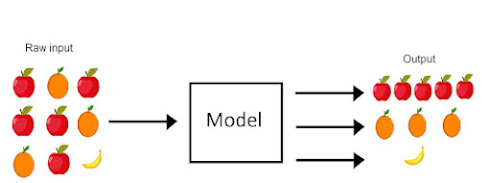Classification Example

Classification is the process of categorizing a given set of data into classes. The data can be categorized into two or more classes.

In machine learning, classification is a supervised learning technique meaning the computer is provided with a set of training data, which contains input data and the corresponding output. The computer learns from this training data and is then able to classify new data into the correct output category.

Classification is one of the most popular techniques in machine learning that is used to solve many real-world problems such as facial recognition, spam detection, medical diagnosis, and many more.

## Top 5 Classification Algorithms

### 1. Logistic Regression

Logistic regression is a powerful machine learning algorithm that can be used for a variety of classification tasks. It is a fast and efficient algorithm that can be trained on large datasets. Logistic regression is also a relatively simple algorithm, which makes it easy to interpret and explain.

It is a supervised learning algorithm that is used to predict the probability of a binary outcome. The outcome is either 1 (positive) or 0 (negative).

Logistic Regression finds the best linear combination of features to separate the two classes. This can be done by using the most popular sigmoid function. The sigmoid function is used to map the real values into a probability between 0 and 1.

Logistic Regression can be also used for multiclass classification which is known as Multinomial Logistic Regression or Softmax Regression. The only difference between these two types of regression is that in the case of multinomial logistic regression, you have more than two classes. For example, let’s say you want to predict if a person will buy your product or not (0) or if they will buy your product in the next 6 months (1). In such a case, you would use multinomial logistic regression.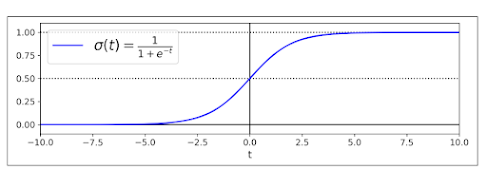Logistic Regression

Applications of Logistic Regression
• Predicting the probability of breast cancer (binary classification)
• Predicting weather conditions (binary classification)
• Stock market prediction (binary classification)
• Sentiment analysis
• Detecting fraudulent credit card transactions
• Pima Indians Diabetes data set (predict whether a person has diabetes or not)

### 2. Naive Bayes Classifier

A Naive Bayes classifier is one of the popular machine learning classification algorithms. It is a simple probabilistic model that is based on the famous Bayes Theorem to predict probabilities for unseen data based on training data

The model can be easily understood by considering an example: Suppose we have a training set consisting of 2 classes - 'spam' and 'not spam. We are given features like word frequencies, email addresses, etc., for both these classes. Based on our training set, we can predict what will be the probability of a new email being spam or not spam using a naive Bayes classifier algorithm.

Naive Bayes is often used in text classification problems. It can be used to predict the class of a new document, given a set of training documents. Each document is represented as a vector of words. The classifier computes the probability of each class, given the document vector. The class with the highest probability is predicted as the class of the new document.

Applications of Naive Bayes Classifier
• Predicting spam emails
• Sentiment analysis in social media platforms
• Classification of diseases based on symptoms
• Predicting movies that a user will like
• Document classification in email marketing systems
• Credit card fraud detection
• Sentiment analysis of tweets (based on emotions)
• Classifying news articles into different categories like sports, entertainment, etc...

### 3. K-Nearest Neighbors

K-Nearest Neighbors or simply KNN is a non-parametric supervised learning algorithm that can be used for both classifications as well as regression tasks. The algorithm is used to find the nearest neighbors of a given data point in the training data set. Once the neighbors are found, the algorithm can then predict the label or value of the data point based on the labels or values of its neighbors.

KNN is a very simple algorithm and is easy to implement. It is also one of the most used algorithms for both classification and regression tasks. The algorithm is also very effective for high-dimensional data sets.

Sometimes KNN is also called a lazy learner algorithm since it does not learn a model from the training data. Instead, it simply uses the training data to make predictions. It uses the training data set to find the nearest neighbors of a given data point and then predicts the label or value of the data point based on the labels or values of its neighbors.

This can be both good and bad depending on the situation. It is good because the algorithm is very simple and easy to implement. It is bad because the algorithm is not very efficient and can be slow for large data sets.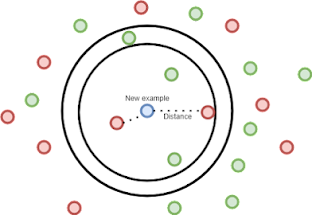K-Nearest

Applications of KNN:

K-Nearest Neighbors is commonly used in Fraud Detection, where it can be used to detect a fraudulent transaction based on similar transactions that have occurred previously. The algorithm can also be used to detect anomalies in data by comparing it with similar datasets. For example, KNN could be used to identify if a bank account is being used for fraudulent activities by analyzing similar accounts that have been flagged as being fraudulent.

### 4. Random Forest Classifier

When you have a lot of variables and need accuracy, Random Forest Classifier is your best friend. It is an ensemble learner (part of a class of algorithms called Boosting) which means it’s made up of several decision trees, allowing it to be more accurate than most single-tree learning algorithms. A forest classifier doesn’t always perform as well as algorithms like SVM or logistic regression when accuracy isn’t your top priority; it does, however, give you better speed/accuracy tradeoffs than most other learning algorithms.

The Random Forest Classifiers are capable of handling multiple classes directly. It is also quite efficient in terms of memory usage, which makes it a good choice for problems where training sets are very large. This algorithm can be used for both regression and classification problems.

It can handle both numerical and categorical variables. To use it on a dataset, you must convert all your features into numerical format (usually by discretizing them). However, if you have many categorical variables that take only two values (e.g., Yes/No), then it’s better to use Logistic Regression instead as it will be more accurate than Random Forest Classifier on such datasets.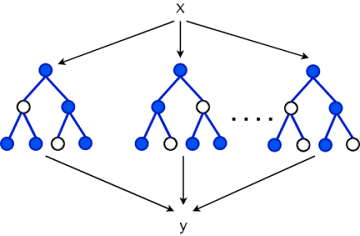Random Forest

Applications of Random Forest Classifier
• Image recognition in image processing (supervised machine learning)
• Face detection in images (supervised machine learning)
• Classification of handwritten digits (supervised machine learning)
• Natural language processing (NLP), such as text classification, authorship attribution, etc.(unsupervised machine learning)

### 5. Support Vector Machine

When comes to classification, one that cannot be avoided is the Support Vector Machine Algorithm. Support Vector Machines(SVM) is a popular and very powerful machine learning algorithm capable of performing both regression and classification.

It works by finding an optimal hyperplane that separates two classes with maximum margin. It simply finds the support vectors and tries to maximize the distance between the two classes. Moreover, SVM can be used to solve binary classification problems as well as multi-class classification problems.

It can be also very powerful because of something known as Kernel Trick. kernel trick is used to find the hyperplane in higher dimensional space. This is done by mapping the data into higher dimensional space using a kernel function. Commonly used kernel functions are linear, polynomial, and radial basis function(RBF) kernels.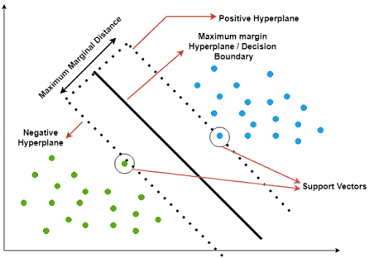Support Vector Machine

Applications of SVM
• It is used in image recognition, bioinformatics, natural language processing, etc.
• It is used in data mining to create models that are useful for classification or regression problems.
• It can be used to predict a numerical value based on a set of input variables.
• It can be used to find patterns in data sets by using techniques like principal component analysis (PCA).